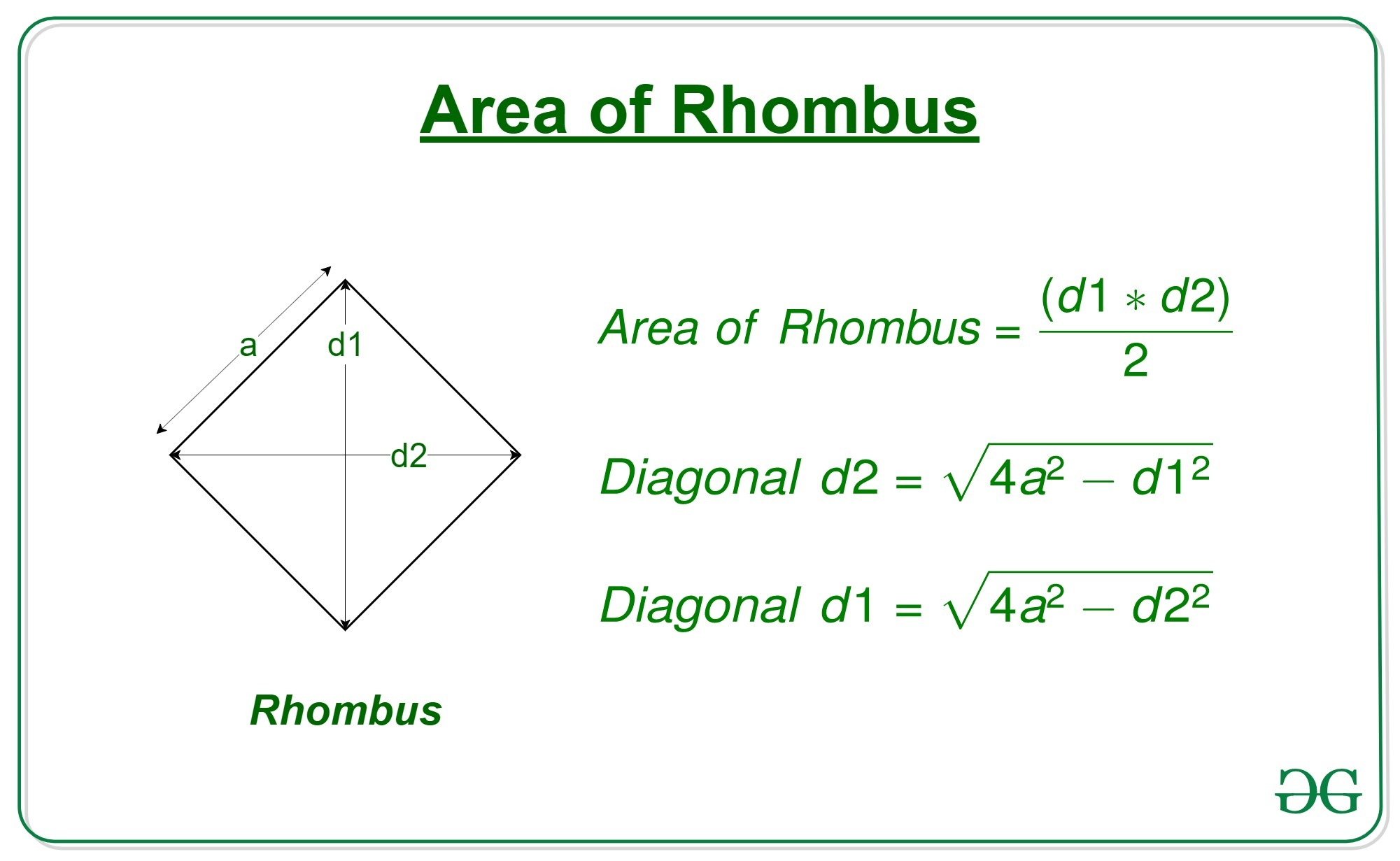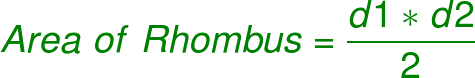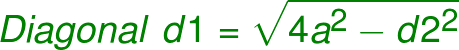Related Articles

# Program to calculate area of a rhombus whose one side and diagonal are given

• Difficulty Level : Expert
• Last Updated : 07 Apr, 2021

Given the length of diagonal ‘d1’ of a rhombus and a side ‘a’, the task is to find the area of that rhombus.

A rhombus is a polygon having 4 equal sides in which both the opposite sides are parallel, and opposite angles are equal.Examples:

```Input: d = 15, a = 10
Output: 99.21567416492215

Input: d = 20, a = 18
Output: 299.3325909419153```

Approach:

• Get the diagonal ‘d1’ and side ‘a’ of the rhombus
• We know that,•
• But since we don’t know the other diagonal d2, we cannot use this formula yet
• So we first find the second diagonal d2 with the help of d1 and a•
• Now we can use the area formula to compute the area of the Rhombus

## C++

 `// C++ program to calculate the area of a rhombus``// whose one side and one diagonal is given``#include``using` `namespace` `std;` `// function to calculate the area of the rhombus``double` `area(``double` `d1, ``double` `a)``{``    ` `    ``// Second diagonal``    ``double` `d2 = ``sqrt``(4 * (a * a) - d1 * d1);` `    ``// area of rhombus``    ``double` `area = 0.5 * d1 * d2;` `    ``// return the area``    ``return` `area;``}` `// Driver code``int` `main()``{``    ``double` `d = 7.07;``    ``double` `a = 5;``    ``printf``(``"%0.8f"``, area(d, a));``}` `// This code is contributed by Mohit Kumar`

## Java

 `// Java program to calculate the area of a rhombus``// whose one side and one diagonal is given``class` `GFG``{` `    ``// function to calculate the area of the rhombus``    ``static` `double` `area(``double` `d1, ``double` `a)``    ``{``        ` `        ``// Second diagonal``        ``double` `d2 = Math.sqrt(``4` `* (a * a) - d1 * d1);``    ` `        ``// area of rhombus``        ``double` `area = ``0.5` `* d1 * d2;``    ` `        ``// return the area``        ``return` `area;``    ``}``    ` `    ``// Driver code``    ``public` `static` `void` `main (String[] args)``    ``{``        ``double` `d = ``7.07``;``        ``double` `a = ``5``;``        ``System.out.println(area(d, a));``    ``}``}` `// This code is contributed by AnkitRai01`

## Python3

 `# Python program to calculate``# the area of a rhombus``# whose one side and``# one diagonal is given` `# function to calculate``# the area of the rhombus``def` `area(d1, a):``    ` `    ``# Second diagonal``    ``d2 ``=` `(``4``*``(a``*``*``2``) ``-` `d1``*``*``2``)``*``*``0.5``    ` `    ``# area of rhombus``    ``area ``=` `0.5` `*` `d1 ``*` `d2``    ` `    ``# return the area``    ``return``(area)` `# driver code``d ``=` `7.07``a ``=` `5``print``(area(d, a))`

## C#

 `// C# program to calculate the area of a rhombus``// whose one side and one diagonal is given``using` `System;` `class` `GFG``{` `    ``// function to calculate the area of the rhombus``    ``static` `double` `area(``double` `d1, ``double` `a)``    ``{``        ` `        ``// Second diagonal``        ``double` `d2 = Math.Sqrt(4 * (a * a) - d1 * d1);``    ` `        ``// area of rhombus``        ``double` `area = 0.5 * d1 * d2;``    ` `        ``// return the area``        ``return` `area;``    ``}``    ` `    ``// Driver code``    ``public` `static` `void` `Main (String []args)``    ``{``        ``double` `d = 7.07;``        ``double` `a = 5;``        ``Console.WriteLine(area(d, a));``    ``}``}` `// This code is contributed by Arnab Kundu`

## Javascript

 ``
Output:
`24.999998859949972`

Attention reader! Don’t stop learning now. Get hold of all the important DSA concepts with the DSA Self Paced Course at a student-friendly price and become industry ready.  To complete your preparation from learning a language to DS Algo and many more,  please refer Complete Interview Preparation Course.

In case you wish to attend live classes with experts, please refer DSA Live Classes for Working Professionals and Competitive Programming Live for Students.

My Personal Notes arrow_drop_up